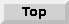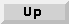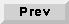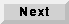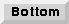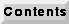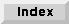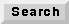## 13.5 Dynamic constructs in CG domain

All multiprocessor code generation domains included in previous releases assumed that the dataflow graph is synchronous (or SDF)-that is, the number of tokens consumed and produced by each star does not vary at run time. We also assumed that the relative execution times of blocks was specified, and did not allow blocks with dynamic behavior, such as the case construct, data-dependent iteration, and recursion. In simulation, however, data-dependent behavior was supported by the DDF (Dynamic Dataflow) domain. The current release allows data-dependent constructs in the code generation domains, by a new clustering technique and a new scheduler called the CGDDF scheduler1.

### 13.5.1 Dynamic constructs as a cluster

Dynamic construct are specified using predefined graph topologies. For example, an if-then-else construct is represented as a galaxy that consists of two DDF stars, `Case` and `EndCase`, and two SDF galaxies to represent the bodies of the `TRUE` or `FALSE` branches. The dynamic constructs supported by the CGDDF scheduler are case, for, do-while, and recursion. The case construct is a generalization of the more familiar if-then-else construct. The topology of the galaxy is matched against a set of pre-determined topologies representing these dynamic constructs.

### 13.5.2 Quasi-static scheduling of dynamic constructs

We treat each dynamic construct as a special SDF star and use a static scheduling algorithm. This SDF star is special in the sense that it may need to be mapped onto more than one processor, and the execution time on the assigned processor may vary at runtime (we assume it is fixed when we compute the schedule). The scheduling results decide the assignment to and ordering of blocks on the processors. At run time, we will not achieve the performance expected from the compile time schedule, because the dynamic constructs behave differently to the compile-time assumptions. The goal of the CGDDF scheduler is to minimize the expected makespan of the program graph at run time.

constructType (`STRING`) Default =
There is no default, the initial value is the value of the galaxy parameter.
Type of the dynamic construct. Must be one of `case`, `for`, `doWhile`, or `recur` (case insensitive).
paramType (`STRING`) Default = `geometric`Type of the distribution. Currently, we support `geometric` distribution, `uniform` distribution, and a `general` distribution specified by a table.
paramGeo (`FLOAT`) Default = `0.5`Geometric constant of a geometric distribution. Its value is effective only if the geometric distribution is selected by paramType. If constructType is `case`, this parameter indicates the probability of branch 1 (the TRUE branch) being taken. If there are more than two branches, use paramFile to specify the probabilities of taking each branch.
paramMin (`INT`) default = `1`Minimum value of the uniform distribution, effective only when the `uniform` distribution is chosen.
paramMax (`INT`) default = `10`Maximum value of the uniform distribution, effective only when the `uniform` distribution is chosen.
paramFile (`STRING`) default = `defParams`The name of a file that contains the information on the general distribution. If the construct is a case construct, each line contains the probability of taking a branch (numbered from 0). Otherwise, each line contains the integer index value and the probability for that index. The indices should be in increasing order.
Based on the specified run-time behavior distribution, we determine the compile-time profile of each dynamic construct. The profile consists of the number of processors assigned to the construct and the (assumed) execution times of the construct on the assigned processors. Suppose we have a for construct. If the loop body is scheduled on one processor, it takes 6 time units. With two processors, the loop body takes 3 and 4 time units respectively. Moreover, each iteration cycle can be paralleled if skewed by 1 time unit. Suppose there are four processors: then, we have to determine how many processors to assign to the construct and how many times the loop body will be scheduled at compile time. Should we assign two processors to the loop body and parallelize two iteration cycles, thus taking all 4 processors? Or should we assign one processor to the loop body and parallelize three iteration cycles, thus taking 3 processors as a whole? The CGDDF scheduler uses a systematic approach based on the distribution to answer these tricky scheduling problems [Ha92]. We can manually determine the number of assigned processors by defining a fixedNum galaxy parameter. Note that we still have to decide how to schedule the dynamic construct with the given number of processors. The Gantt chart display will show the profile of the dynamic construct.

### 13.5.3 DDF-type Stars for dynamic constructs

A code generation domain should have DDF stars to support dynamic constructs with the CGDDF scheduler. For example, the `Case` and `EndCase` stars are used in the case, do-while, and recursion constructs, which differ from each other in the connection topology of these DDF stars and SDF galaxies. Therefore, if the user wants to use one of the above three dynamic constructs, there is no need to write a new DDF star. Like a DDF star, the `Case` star has dynamic output portholes as shown in the `CGCCase.pl` file. For example:

` outmulti {	name { output }	type { =input }	num { 0 }}`
The for construct consists of an UpSample type star and a DownSample type star, where UpSample and DownSample are not the star names but the types of the stars: if a star produces more than it consumes, it is called an UpSample star. In the preprocessor file, we define a method `readTypeName`, as shown below.

`method {	name { readTypeName }	access { public }	type { "const char *" }	code { return "UpSample"; }}`
Examples of UpSample type stars are `Repeater` and `DownCounter`. These stars have a data input and a control input. The number of output data tokens is the value of the integer control input, and is thus data-dependent. Conversely, we can design a DownSample star that has the following method:

`method {	name { readTypeName }	access { public }	type { "const char *" }	code { return "DownSample"; }}`
Examples of DownSample type stars are `LastOfN`, and `SumOfN`. These stars have a data input and a control input. The number of input tokens consumed per invocation is determined by the value of the control input.1 In version 0.4 of Ptolemy, dynamic constructs were supported with a separate domain called the CGDDF domain. We have since designed a mechanism for wormhole interfaces to support the CGDDF domain inside the CG domain. By using clustering instead of wormholes, we were able to clean up the code significantly in this release